# Fifty years of the renormalization group

29 August 2001

Renormalization was the breakthrough that made quantum field theory respectable in the late 1940s. Since then, renormalization procedures, particularly the renormalization group method, have remained a touchstone for new theoretical developments. Distinguished theorist Dmitry Shirkov, who worked at the cutting edge of the field with colleague Nikolai Bogoliubov, relates the history of the renormalization group.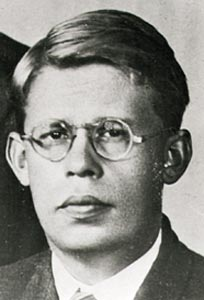Nicolai Bogoliubov
Quantum field theory is the calculus of the microworld. It consists principally of a combination of quantum mechanics and special relativity, and its main physical ingredient – the quantum field – brings together two fundamental notions of classical (and non-relativistic quantum) physics – particles and fields.

For instance, the quantum electromagnetic field, within appropriate limits, can be reduced to particle-like photons (quanta of light), or to a wave process described by a classical Lorentz field. The same is true for the quantum Dirac field.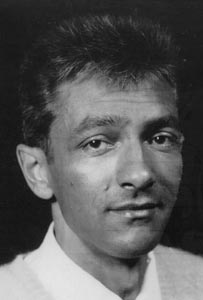André Petermann
Quantum field theory (QFT) , as the theory of interacting quantum fields, includes the remarkable phenomenon of virtual particles, which are related to virtual transitions in quantum mechanics. For example, a photon propagating through empty space (the classical vacuum) undergoes a virtual transition into an electron-positron pair. Usually, this pair undergoes the reverse transformation: annihilation back into a photon. This sequence of two transitions is known as the process of vacuum polarization (figure 1(a)). Hence the vacuum in QFT is not an empty space; it is filled by virtual particle-antiparticle pairs.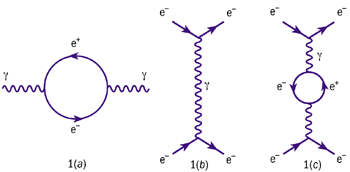(a) A photon propagating through empty space (the classical vacuum) undergoes a virtual transition into an electron-positron pair. Feynman diagrams are the classical method for displaying such processes. (b) and (c) show such diagrams for the scattering of an electron and a proton.
Another example of vacuum polarization is the electromagnetic interaction between two electric charges (e.g. between two electrons, or between a proton and an electron). In QFT, rather than a Coulomb force described by a potential, the interaction corresponds to an exchange of virtual photons, which, in turn, propagate in space-time accompanied by virtual electron-positron pairs (figure 1(c)). The theory of the interaction of quantum fields of radiation (photons) and of quantum Dirac fields (electrons and positrons) formulated in the early 1930s is known as quantum electrodynamics.

QFT calculation usually results in a series of terms, each of which represents the contribution of different vacuum-polarization mechanisms (illustrated by Feynman diagrams). Unfortunately, most of these terms turn out to be infinite. For example, electron-proton scattering, as well as Feynman diagram 1(b) (Møller scattering), also includes radiative corrections (figure 1(c)). This last contribution is infinite, owing to a divergence of the integral in the low wavelength/high-energy region of possible momentum values of the virtual electron-positron pair. One such infinity is the analogue of the well known infinite self-energy of the electron in classical electrodynamics.

When theorists met this problem in the 1930s, they were puzzled – the first QED approximation (e.g. for Compton scattering) produces a reasonable result (the Klein-Nishina-Tamm formula), while the second, involving more intricate vacuum-polarization effects, yields an infinite contribution.

### Renormalization is discovered

The puzzle was resolved in the late 1940s, mainly by Bethe, Feynman, Schwinger and Dyson. These famous theoreticians were able to show that all infinite contributions can be grouped into a few mathematical combinations, Zi (in QED, i = 1,2), that correspond to a change of normalization of quantum fields, ultimately resulting in a redefinition (“renormalization”) of masses and coupling constants. Physically, this effect is a close analogue of a classical “dressing process” for a particle interacting with a surrounding medium.

The most important feature of renormalization is that the calculation of physical quantities gives finite functions of new “renormalized” couplings (such as electron charge) and masses, all infinities being swallowed by the Z factors of the renormalization redefinition. The “bare” values of mass and electric charge do not appear in the physical expression. At the same time the renormalized parameters should be related to the physical ones, measured experimentally.

When suitable renormalized quantum electrodynamics calculations gave results that were in precise agreement with experiment (e.g. the anomalous magnetic moment of the electron, where agreement is of the order of 1 part in 10 billion), it was clear that renormalization is a key prerequisite for a theory to give useful results.

Once the field theory infinities have been suitably excluded, the resultant finite parameters have the arbitrariness that corresponds to the possibility of various experimental measurements. For example, the electric charge of the electron measured at the Z mass (at CERN’s LEP electron-positron collider) yields the fine structure constant a as 1/128.9 (the value used in the theoretical analysis of LEP events), rather than the famous Millikan value 1/137. However, the theoretical expressions for physical quantities, like observed cross-sections, should be the same, invariant with respect to renormalization transformations equivalent to the transition from one a value to the other. In the hands of astute researchers, this invariance with respect to arbitrariness has been developed into one of the most powerful techniques of mathematical physics. (For a more technically detailed historical overview, see Shirkov 1993.)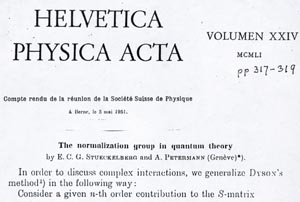This groundbreaking two-page note by Ernest Stückelberg and André Petermann in 1951, entitled “The normalization group in quantum theory”, went unnoticed.
The impressive story of an elegant mathematical method that is now widely used in various fields of theoretical and mathematical physics started just half a century ago. The first published “signal” – a two-page note by Ernest Stückelberg and André Petermann (1951), entitled “The normalization group in quantum theory” (figure 2) remained unnoticed, even by QFT experts.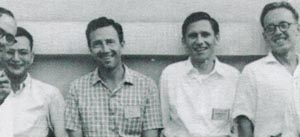Group photo at the 1959 high-energy physics “Rochester” conference in Kiev. Left to right: Stanley Mandelstam (half obscured); Murray Gell-Mann; Francis Low; Harry Lehmann; and Dimitry Shirkov.
However, from the mid-1950s the Renormalization Group Method to improve approximate solutions to QFT equations became a powerful tool for investigating singular behaviour in both the ultraviolet (higher energy) and infrared (lower energy) limits.

Later, this method was transferred from QFT to quantum statistics for the analysis of phase transitions and then to other fields of theoretical and mathematical physics.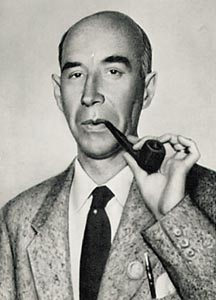Ernest C G Stückelberg
In their next major article (Stückelberg & Petermann 1953), the same authors gave a clearer formulation of their discovery. They distinctly stated that, in QFT, finite renormalization transformations form a continuous group – the Lie group – for which differential Lie equations hold. Unfortunately, the paper was published in French, a language not very popular among theorists at that time. In any case, it was not mentioned in Murray Gell-Mann and Francis Low’s important paper of 1954.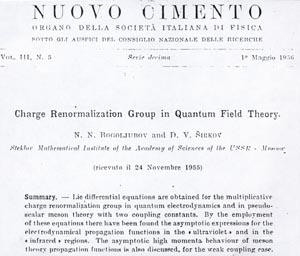The term “Renormalization Group” was first introduced in pioneering papers by Nicolai Bogoliubov and Dmitry Shirkov in the mid-1950s.
A more complete and transparent picture appeared in 1955-1956 with papers by Nicolai Bogoliubov and Dmitry Shirkov. In two short Russian-language notes (Bogoliubov & Shirkov 1955a), these authors established a connection between the work of Stückelberg and Petermann and that of Gell-Mann and Low, and they devised a simple algorithm, the Renormalization Group Method (RGM – using differential group equations and the famous beta-function) for practical analysis of ultraviolet and infrared asymptotics. These results were soon published in English (Bogoliubov & Shirkov 1956a, 1956b) and then included in a special chapter of a monograph (Bogoliubov & Shirkov 1959), and from that time the RGM became an indispensable tool in the QFT analysis of asymptotic behaviour.Momentum transfer evolution of QED effective electron charge squared. The monotonically rising theoretical curve is confronted with precise measurements at the Z mass at CERN's LEP electron-positron collider.
It was in these papers that the term “Renormalization Group” was first introduced (figure 3), as well as the central notion of the RGM algorithm – an invariant (or effective, or running) coupling. In QED, this function is just a Fourier transform of the effective electron charge squared, e2(r), first introduced by Dirac (1934).
The physical picture qualitatively corresponds to the classical electric charge, Q, inserted into polarizable media, such as electrolytes. At a distance r from the charge, due to polarization of the medium, its Coulomb field will depend on a function Q(r) – the effective charge – instead of a fixed quantity, Q. In QED, polarization is produced by vacuum quantum fluctuations. Figure 4 shows the momentum transfer evolution of QED effective coupling (a = e2/hc).

### Applications in QFT

The very first applications of the RGM included the infrared and ultraviolet asymptotic analysis as well as the resolution (Bogoliubov & Shirkov 1955b) of the “ghost-problem” for renormalizable local QFT models.Renormalization in action – the quantitative correspondence between theoretical curves for effective QCD coupling squared, a s (Q2), and data (taken from the recent Particle Data Group review).
The most important physical result obtained via RGM was the theoretical discovery (Gross & Wilczek 1973; Politzer 1973) of the “asymptotic freedom” of non-Abelian vector models. In contradistinction with QED, here the vacuum polarization effect has an opposite sign owing to fluctuations of non-Abelian vector mesons, such as gluons. This explained quantitatively why quarks interacted less at smaller distances, and it became a cornerstone of the theoretical QFT now known as Quantum Chromodynamics (QCD; figure 5).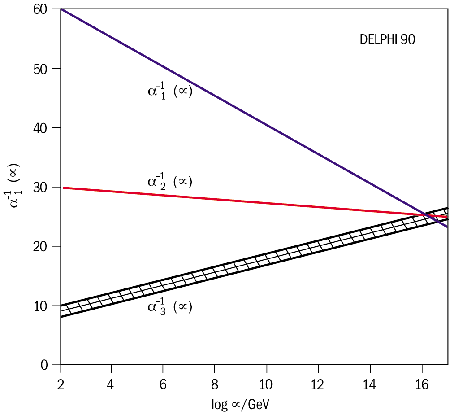Early results from CERN's LEP electron-positron collider showed how varying (running) coupling constants support the idea of the Grand Unification of strong and electromagnetic and weak interactions.
Another illustration, this time more speculative, is the so-called “chart of interaction” that gave rise to the idea of the Grand Unification of strong and electroweak interactions.

At the beginning of the 1970s, Kenneth Wilson (1971) devised a specific version of the RG formalism for statistical systems. It was based on Kadanoff’s idea of “blocking”; more specifically, averaging over a small part of a big system. Mathematically, the set of blocking operations forms a discrete semigroup, different from that of QFT. The Wilson group was then used for the calculation of critical indices in phase transitions. As well as critical phenomena (in the 1970s and 1980s), it was applied to polymers, percolation, non-coherent radiation transfer, dynamical chaos and some other problems. A rather transparent motivation of Wilson’s RG facilitated this expansion. Kenneth Wilson was awarded the 1982 Nobel Prize for this work.

On the other hand, in the 1980s a more simple and general formulation of the QFT renormalization group was found (Shirkov 1982, 1984). This relates the RG symmetry to a widely known notion of mathematical physics – self-similarity. Here, the RG symmetry appears in the role of symmetry of a particular solution with respect to its reparameterization transformation. It can be treated as a functional generalization of self-similarity – functional similarity.

Later, this formulation was successfully applied to some boundary value problems of mathematical physics, such as to the problem of a self-focusing laser beam in nonlinear media (Kovalev & Shirkov 1997). Here, the RG-type symmetry solution is described by a multiparametric group, and it enables the two-dimensional structure of the solution singularity to be studied.

### Further reading

N N Bogoliubov and D V Shirkov 1955a Dokl. Akad. Nauk.SSSR 103 203; ibid 391 (in Russian). For a short review in English, see F Dyson 1956 Math. Rev. 17 441.

N N Bogoliubov and D V Shirkov 1955b Dokl. Akad. Nauk. SSSR 105 685 (in Russian). For a short review in English see F Dyson 1956 Math. Rev. 17 1033.

N N Bogoliubov and D V Shirkov 1956a Nuovo Cim. 3 845-63.

N N Bogoliubov and D V Shirkov1956b Sov. Phys. JETP 3 57.

N N Bogoliubov and D V Shirkov 1959 Introduction to the theory of quantized fields (Wiley-Interscience, New York).

P A M Dirac 1934 Theorie du positron 7-eme conseil du physique Solvay (Gautier-Villars, Paris) 203-230.

M Gell-Mann and F E Low 1954 Phys. Rev. 95 1300.

D Gross and F Wilczek 1973 Phys. Rev. Lett. 30 1343.

H D Politzer 1973 Phys. Rev. Lett. 30 1346.

V F Kovalev and D V Shirkov 1997 J. Nonlin. Optics and Mater. 6 443.

D V Shirkov 1982 Sov. Phys. Dokl. 27 197.

D V Shirkov 1984 Teor. Mat. Fiz. 60 778.

D V Shirkov 1993 Historical Remarks on the Renormalization Group Renormalization from Lorentz to Landau (and Beyond) ed. Laurie M Brown (Springer-Verlag, New York) 167-186.

E Stückelberg and A Petermann 1951 Helv. Phys. Acta 24 317.

E Stückelberg and A Petermann 1953 Helv. Phys. Acta 26 499 (in French).

K Wilson 1971 Phys. Rev. B4 3174, 3184.